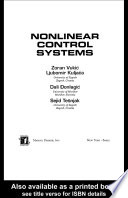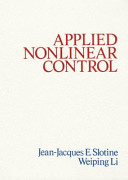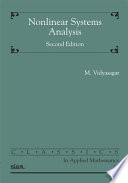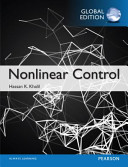### Nonlinear Control Systems

#### Course Description

Dynamic effects due to nonlinearities in systems. Common nonlinear elements in control systems. Phase-plane analysis. Linearization of nonlinear systems. Harmonic linearization. Describing function. Self-oscillations (limit-cycles). Forced oscillations. Stability of nonlinear systems. Feedback linearization. Sliding mode control.

#### Learning Outcomes

1. recognize common nonlinear control problems
2. apply some powerfull analysis methods
3. apply some practical design methods
4. discover how nonlinearities can improve system dynamics
5. apply stability analysis methods to real systems
6. identify and remove negative effects that appear in nonlinear control systems

#### Forms of Teaching

Lectures

Three hours of lectures per week.

Laboratory

Four laboratory exercises.

Continuous Assessment Exam
Laboratory Exercises 0 % 20 % 0 % 20 %
Mid Term Exam: Written 0 % 20 % 0 %
Final Exam: Written 0 % 20 %
Final Exam: Oral 40 %
Exam: Written 0 % 40 %
Exam: Oral 40 %
##### Comment:

The condition for taking the oral exam is that all laboratory exercises have been completed and that 50% of the maximum sum of points from laboratory exercises, midterm exam and final exam has been achieved.

#### Week by Week Schedule

1. Introduction. Examples of nonlinear control systems. Characteristic nonlinear behaviours.
2. Dynamic analysis of nonlinear systems. Typical nonlinear elements.
3. Phase portrait of nonlinear systems.
4. Tangent method of linearization. Secant method of linearization. Harmonic linearization.
5. Describing function
6. Self-oscillations
7. Self-oscillations - examples.
8. Midterm exam
9. Stability of self-oscillations
10. Forced oscillations
11. Feedback linearization. Controllability of nonlinear systems.
12. Lyapunov stability in nonlinear control systems (algebraic criteria)
13. Lyapunov stability for nonlinear time-varying systems. Sliding mode control
14. Popov stability (graphical criteria)
15. Final exam

#### Study Programmes

Control Engineering and Automation (profile)
Theoretical Course (2. semester)

#### LiteratureZ. Vukić, Lj. Kuljača, D. Đongalić, S. Tešnjak (2003.), Nonlinear Control Systems, CRC PressJean-Jacques E. Slotine, Weiping Li (1991.), Applied Nonlinear Control,M. Vidyasagar (2002.), Nonlinear Systems Analysis, SIAMHassan K. Khalil (2014.), Nonlinear Control,

#### General

ID 222513
Winter semester
5 ECTS
L1 English Level
L1 e-Learning
45 Lectures
8 Laboratory exercises